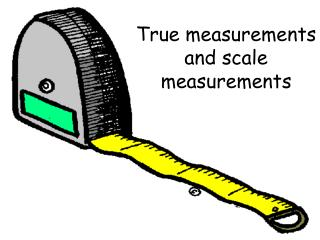DownloadDownload PresentationTrue measurements and scale measurements

# True measurements and scale measurements

Download Presentation## True measurements and scale measurements

- - - - - - - - - - - - - - - - - - - - - - - - - - - E N D - - - - - - - - - - - - - - - - - - - - - - - - - - -
##### Presentation Transcript

1. True measurements and scale measurements

2. Why do we need to use scale to draw objects or house plans etc.? We couldn’t possibly fit the true measurements of a new house or a new design for a playground onto a piece of paper.

3. What jobs is this skill important for? Construction workers Architect Drafters

4. How do we use scale measurements? It is important that the drawing is measured accurately using the scale. The measurements must make an exact copy of the real object/building but in a smaller size like a replica of a car

5. Ready to try some examples?

6. Imagine this house measures 10 cm in length. • The scale used here is 1cm = 2.5m • This means that every centimetre we draw actually represents 2.5 metres length in real life. So if the house measures 10cm in length what is the true length of the house? 10x2.5=25 25m true length

7. Let’s try another one! • The height of the scale drawing of this boat is 23cm. • The scale is 1cm=7m • How will we work out the true height of the boat? • Remember every centimetre is worth 7 metres in real life. • 23x7=161 • True height is 161 metres!